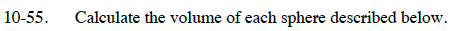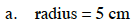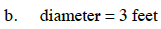Home > CC3 > Chapter Ch10 > Lesson 10.1.4 > Problem10-55

10-55.
1. Calculate the volume of each sphere described below. Homework Help ✎

1. radius = 5 cm

2. diameter = 3 feet$\text{The formula to find the volume}$

$\text{ of a sphere is \textit{V} = \frac{4}{3}}\pi r^3$

Remember the units!Remember to use the radius, not the diameter.